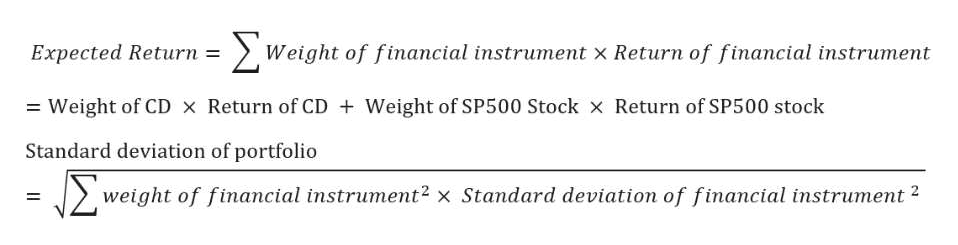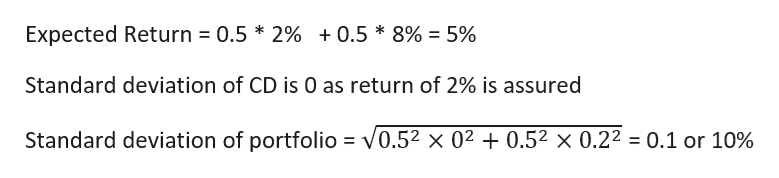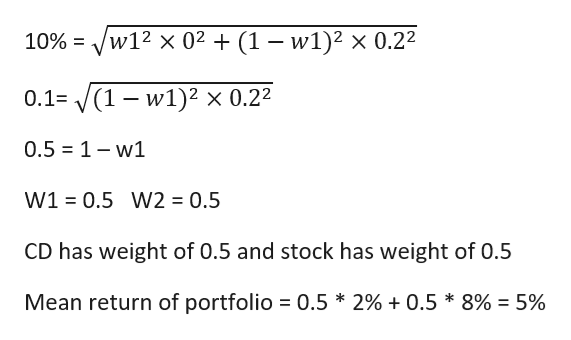# Q5. You are considering two investment choices: a. 1 year CD that pays 2% for sure; b. investing in SP500 with 8% expected and 20% stdev.Q5c. You want to reach 15% mean return per year as a minimum. What is the least amount you need to allocate to stocks? What is the stdev of the portfolio?

Question
9 views

Q5. You are considering two investment choices: a. 1 year CD that pays 2% for sure; b. investing in SP500 with 8% expected and 20% stdev.

Q5c. You want to reach 15% mean return per year as a minimum. What is the least amount you need to allocate to stocks? What is the stdev of the portfolio?

check_circle

Step 1

Portfolio Mean and SD

I am answering all the sub-parts

Q5. You are considering two investment choices: a. 1 year CD that pays 2% for sure; b. investing in SP500 with 8% expected and 20% stdev.

Q5a. If you put 50% in each of the two, what in the mean and stdev for your portfolio return?

Q5b. You want to maximize your expected return for the portfolio, as long as the stdev risk is no more than 10% per year. What is the allocation to CD and to stocks? What is the mean return of the portfolio?

Q5c. You want to reach 15% mean return per year as a minimum. What is the least amount you need to allocate to stocks? What is the stdev of the portfolio?

Formula To calculate  Expected Return and standard deviation of portfoliohelp_outlineImage TranscriptioncloseΣ Weight of financial instrument x Return of financial instrument Expected Return = Weight of SP500 Stock x Return of SP500 stock Weight of CD x Return of CD Standard deviation of portfolio Σ weight of financial instrument2 x Standard deviation of financial instrument 2 fullscreen
Step 2

5A) Calculation of Expected Return and Standard Deviation Of portfolio

Standard deviation of CD is 0 as return of 2% is assuredhelp_outlineImage TranscriptioncloseExpected Return 0.5 * 2% +0.5 * 8% = 5% Standard deviation of CD is 0 as return of 2% is assured Standard deviation of portfolio = V0.52 x 02 +0.52 x 0.22 = 0.1 or 10% fullscreen
Step 3

5B) Let the standard deviation of portfolio be 10%

Let weights of w1 and w2 be assigned to CD and stock respectively.

W1 + w2 = 1 a...help_outlineImage Transcriptionclose/w12 x 02 (1 - w1)2 x 0.22 10% '(1 w1)2 x 0.22 0.1= 0.5 1-w1 W1 0.5 W2 = 0.5 CD has weight of 0.5 and stock has weight of 0.5 Mean return of portfolio 0.5 * 2% + 0.5 * 8% = 5% fullscreen

### Want to see the full answer?

See Solution

#### Want to see this answer and more?

Solutions are written by subject experts who are available 24/7. Questions are typically answered within 1 hour.*

See Solution
*Response times may vary by subject and question.
Tagged in

### Statistics